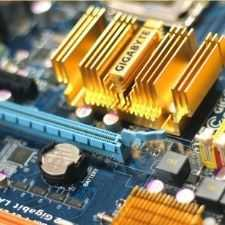Electronics
Community of Electronics Engineers to discuss all concepts, topics, questions and doubts.
612 Members
Join this group to post and comment.danilorj • Mar 4, 2011

# RLC Series Circuit

Guys,
Let's suppose we have a RLC series circuit feeded by tension source initially with no energy stored. When we apply an unitary step of tension at t=0 at the terminals of the circuit, we observe a current of i(t)=(125/24) * exp(-700t)* Sin(2400t) mA that goes around the circuit for t>0s.
I want to find the values of R, L, C.
I was trying to match the laplace transformation of the current above to the laplace transformation of the circuit for the current but no success.danilorj • Mar 4, 2011
Let me explain it better
The Laplace transformation of the given current is I(s)=12500/[(s+700)^2+2400^2]
And if I apply the Kirchhoff Law Tension around the RLC series circuit I get
I(s)=1/[s*(LC*s^2+RC*s+1)]. But I don't know what to do with this. They seem to be different, one has degree 3 and the other has degree 2 in the denominator.danilorj • Mar 4, 2011
And I think the best way of doing this is using the Laplace transformation, cause solving the differential equation would take a very hard work, and I don't think it's gonna work as well.aicbal • Mar 24, 2011
I(s)=12500/[(s+700)^2+2400^2]

the problem is in the above equation. you must mistaken to take laplace transform of unitary step as 1. but it should be 1/s. then your derived above equation contain s^3, then just compare with the standard equation.